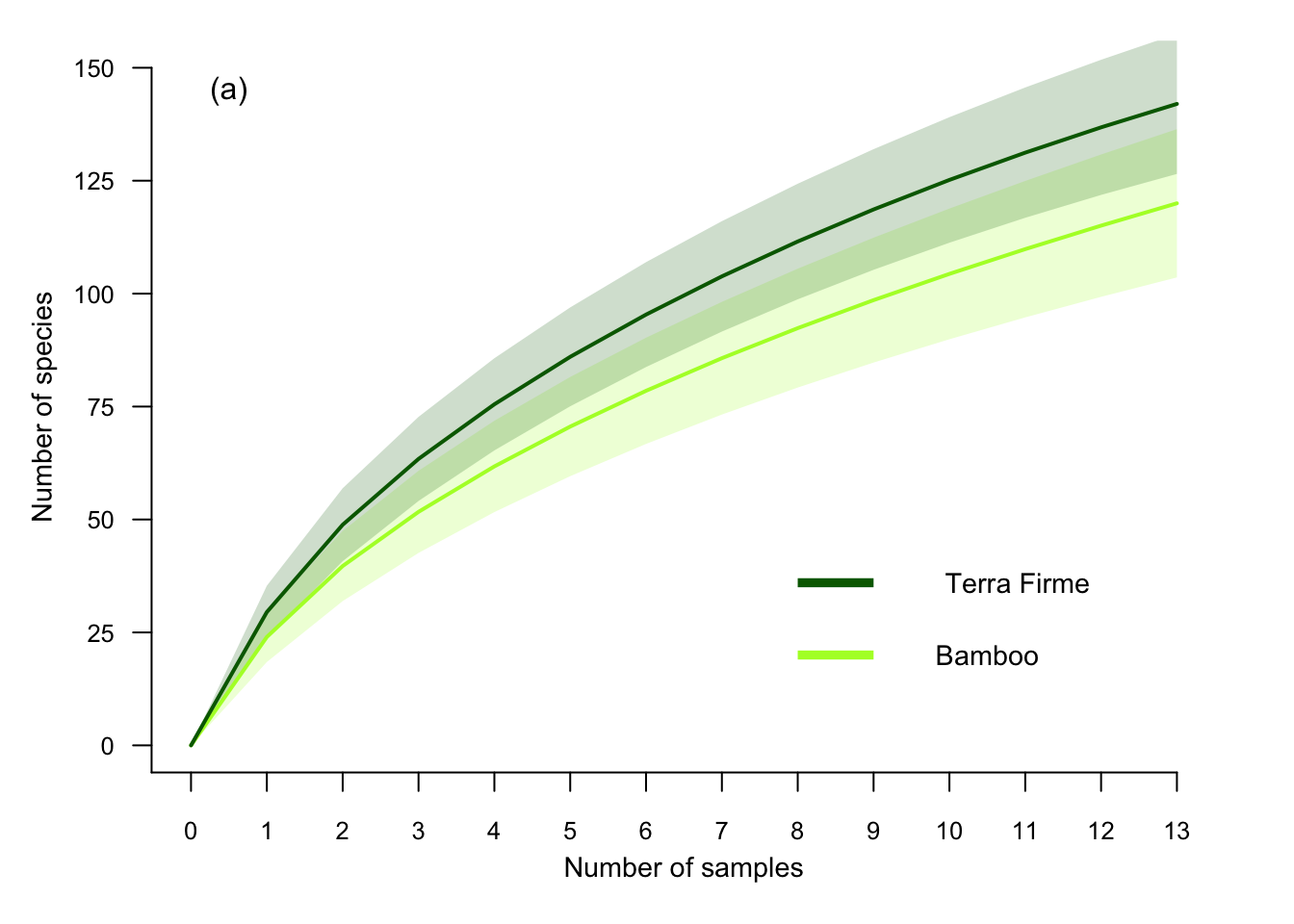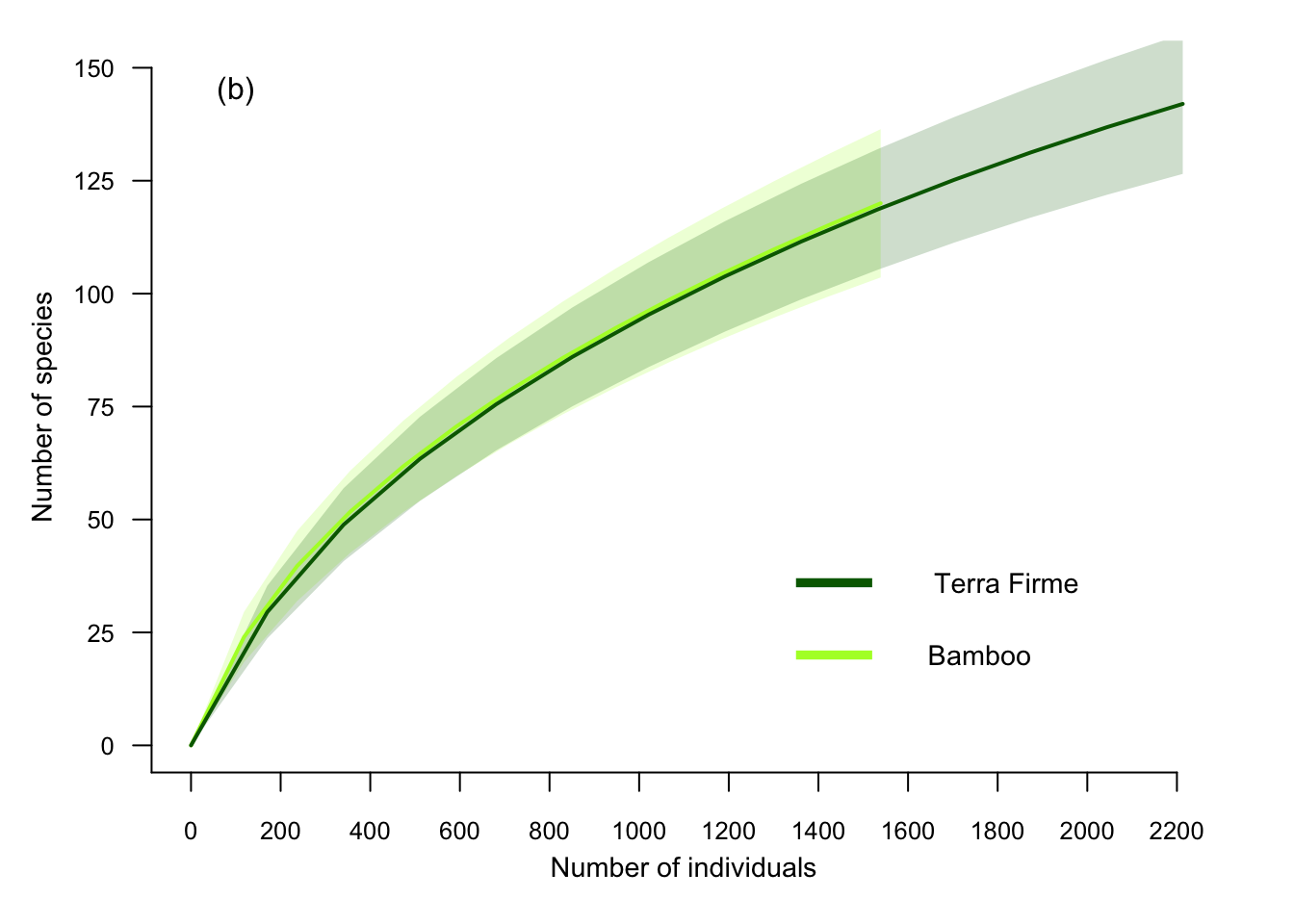Use species abundance data to draw species accumulation curves for beetle species found in bamboo and terra firme forest

# get data
data <- read.csv("http://vonmay.cikeys.com/wp-content/uploads/2020/02/species_accumulation_curves.csv", stringsAsFactors=F)
# check habitat categories
unique(data$Habitat) ##  "Bamboo" "TerraFirme" # subset dataset by habitat bamboo <- data[ data$Habitat == "Bamboo" , ]
terraFirme <- data[  data$Habitat == "TerraFirme" , ] # check range of species in each habitat range(bamboo$Species)
##   24 120
range(terraFirme$Species) ##  29.51 142.00 Plot 1: sample-based and individual-based species accumulation curves # define plot dimensions and layout quartz.options(height=4, width=6) plot.new() par(oma = c(0.1,0.1,0.1,0.1)) par(mar = c(4, 4, 1, 2)) par(cex.axis=0.8) plot.new() plot.window(xlim = c(0, 13), ylim = c(0, 150)) # set up values for bamboo (*Note that a zero was added for both x and y values) xb <- c(c(0,bamboo$Samples), c(rev(bamboo$Samples),0)) yb <- c(c(0,bamboo$Sobs_95_CI_LowerBound), c(rev(bamboo$Sobs_95_CI_UpperBound),0)) # set up values for terraFirme (*Note that a zero was added for both x and y values) xt <- c(c(0,terraFirme$Samples), c(rev(terraFirme$Samples),0)) yt <- c(c(0,terraFirme$Sobs_95_CI_LowerBound), c(rev(terraFirme$Sobs_95_CI_UpperBound),0)) # plot the polygon for each habitat: polygon(x = xb, y = yb, col = rgb(173/255,255/255,47/255, 0.2), border = F) polygon(x = xt, y = yt, col = rgb(0,102/255,0, 0.2), border = F) # add species richness as a line: lb <- lines(c(0,bamboo$Samples), c(0,bamboo$Species), col=rgb(173/255,255/255,47/255), lwd = 2) lt <- lines(c(0,terraFirme$Samples), c(0,terraFirme$Species), col=rgb(0,102/255,0), lw = 2) axis(1, at=seq(-1, 13, by = 1)) axis(2, at=seq(-25, 150, by=25), las=1) mtext("Number of samples", side = 1, line = 2, cex = 0.9) mtext("Number of species", side = 2, line = 2.5, cex = 0.9) #Legend text(x=10.9, y=36, labels="Terra Firme", cex=0.9) polygon(x=c(8,8,9,9), y=c(37, 35, 35, 37), col=rgb(0,102/255,0), border=NA) text(x=10.5, y=20, labels="Bamboo", cex=0.9) polygon(x=c(8,8,9,9), y=c(21, 19, 19, 21), col=rgb(173/255,255/255,47/255), border=NA) # add one more label text(x=0.5, y=145, labels="(a)", cex=1)Plot 2: Individual-based species accumulation curve # check range of number of individuals in each habitat (to adjust plot window) range(bamboo$Individuals)
##   118.38 1539.00
range(terraFirme$Individuals) ##  170.23 2213.00 # define plot dimensions and layout quartz.options(height=4, width=6) plot.new() par(oma = c(0.1,0.1,0.1,0.1)) par(mar = c(4, 4, 1, 2)) par(cex.axis=0.8) plot.new() plot.window(xlim = c(0, 2200), ylim = c(0, 150)) # set up values for bamboo (*Note that a zero was added for both x and y values) xb <- c(c(0,bamboo$Individuals), c(rev(bamboo$Individuals),0)) yb <- c(c(0,bamboo$Sobs_95_CI_LowerBound), c(rev(bamboo$Sobs_95_CI_UpperBound),0)) # set up values for terraFirme (*Note that a zero was added for both x and y values) xt <- c(c(0,terraFirme$Individuals), c(rev(terraFirme$Individuals),0)) yt <- c(c(0, terraFirme$Sobs_95_CI_LowerBound), c(rev(terraFirme$Sobs_95_CI_UpperBound),0)) # plot the polygon for each habitat: polygon(x = xb, y = yb, col = rgb(173/255,255/255,47/255, 0.2), border = F) polygon(x = xt, y = yt, col = rgb(0,102/255,0, 0.2), border = F) # add species richness as a line: lb <- lines(c(0,bamboo$Individuals), c(0,bamboo$Species), col=rgb(173/255,255/255,47/255), lwd = 2) lt <- lines(c(0,terraFirme$Individuals), c(0,terraFirme\$Species), col=rgb(0,102/255,0), lw = 2)

axis(1, at=seq(-200, 2200, by = 200))
axis(2, at=seq(-25, 150, by=25), las=1)

mtext("Number of individuals", side = 1, line = 2, cex = 0.9)
mtext("Number of species", side = 2, line = 2.5, cex = 0.9)

#Legend

text(x=1820, y=36, labels="Terra Firme", cex=0.9)
polygon(x=c(1350,1350,1520,1520), y=c(37, 35, 35, 37), col=rgb(0,102/255,0), border=NA)

text(x=1760, y=20, labels="Bamboo", cex=0.9)
polygon(x=c(1350,1350,1520,1520), y=c(21, 19, 19, 21), col=rgb(173/255,255/255,47/255), border=NA)

text(x=100, y=145, labels="(b)", cex=1)